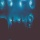Home
IT Knowledge
Inspiration
Languages
EN

# Python - convert list to string

0 points
Created by:Lennox-A
395

In this article, we would like to show you how to convert list to a string in Python.

Quick solution:

``````my_list = ["a", "b", "c"]
text = "".join(my_list)

print(text)  # abc``````

## Practical example

In this example, we convert list to a string using `join()` method with a specified separator. This time as a separator we use a single space (`" "`).

``````my_list = ["dirask", "is", "awesome"]
text = " ".join(my_list)

Output:

``dirask is awesome``

Note:

We use a single space (`" "`) as a separator in `join()` method. To use a different separator, simply type new character in double-quotes before the metod.

``"_".join(my_list)``

Output:

``dirask_is_awesome``